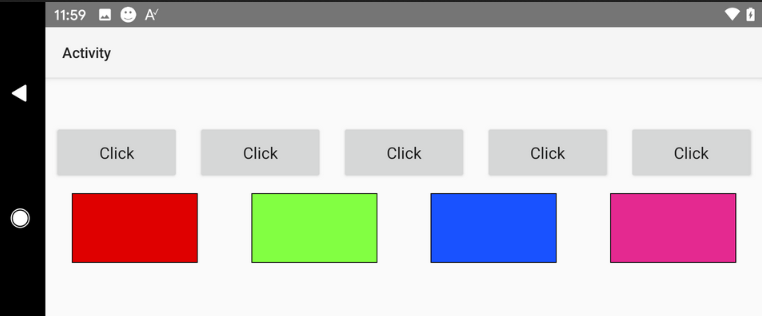# Android Tutorial[B4X] [Visual Designer] Spreading Controls

Question: How can I build a layout with X controls spread horizontally or vertically?

Answer: You can use the designer script for this. As the designer script engine (currently) doesn't support loops it requires copying a few lines, however it is quite simple.

The code goes like this:
B4X:
``````n = 4: MaxSize = 120dip : MinGap = 5dip 'change here (n = number of views)
AllWidth = 100%x
w = Min(AllWidth / n - MinGap, MaxSize)
gap = (AllWidth - n * w) / n

'Only change the views names and 'i' value:
i = 0 : Panel1.SetLeftAndRight((i + 0.5) * gap + i * w,(i + 0.5) * gap + (i + 1) * w)
i = 1 : Panel2.SetLeftAndRight((i + 0.5) * gap + i * w,(i + 0.5) * gap + (i + 1) * w)
i = 2 : Panel3.SetLeftAndRight((i + 0.5) * gap + i * w,(i + 0.5) * gap + (i + 1) * w)
i = 3 : Panel4.SetLeftAndRight((i + 0.5) * gap + i * w,(i + 0.5) * gap + (i + 1) * w)``````

Example is attached.#### Attachments

• 1.zip
9.5 KB · Views: 595

#### hatzisn

##### Well-Known Member
Longtime User
Great. Maybe in next update there could be a loop or auto code generation for this.

Edit - Maybe this (loop) could be done with arrays of controls with reference to the array only in Designer